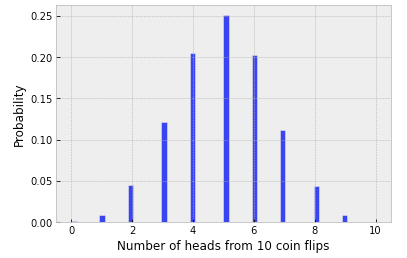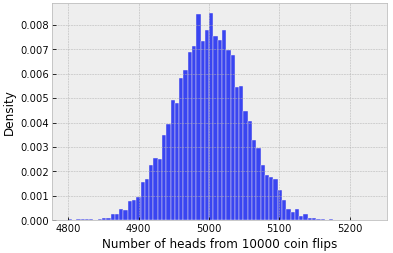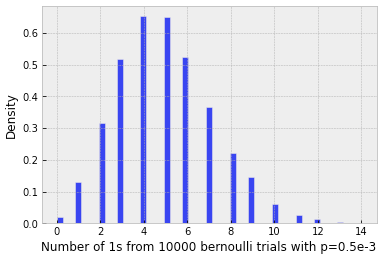# Binomial distribution [discrete]

The binomial distribution is a discrete distribution that describes the probability of getting exactly x successes in n trials of a probability experiment:

where n = the total number of trials, x = the number of successes (1, 2, 3, …, n), p = the probability of success (e.g., getting head in coin flip; user click a link for web activity analysis)

## Example: Probability mass function of getting heads with 10 coin flips from 10000 repeated trials of the experiment

`import scipy.stats# Simulate random variable for the number of heads obtained# from 10 coin flips carried over 10000 repeated experiment trialsr = scipy.stats.binom.rvs(n=10, p=0.5, size=10000, random_state=42)`Figure 1: Probability mass function for the number of heads when flipping 10 fair coins

Concept refresher: What is PMF? PMF stands for probability mass function. It is the probability distribution function for discrete distributions and describes the probability associated with each discrete outcome.

## The mean and standard deviation of a binomial distribution

`binomial_mean = n * p# binomial_mean = 10 * 0.5 = 5binomial_std = np.sqrt(n * p * (1 - p))# binomial_std = np.sqrt(10 * 0.5 * (1 -0.5)) = 1.58`

# Normal distribution [continuous]

Binomial approximation: large n

Despite the continuous vs. discrete distinction between the binomial and normal distributions, once the number of trials — that is, the parameter n — within each round of binomial experiment becomes large enough, binomial distribution becomes a good approximation of the normal distribution.Figure 2: As the number of Bernoulli trials increase within each of the binomial trials, binomial distribution becomes an approximation for the continuous normal distribution
`r = scipy.stats.binom.rvs(n=10000, p=0.5, size=10000, random_state=42)`

# Poisson distribution [discrete]

Binomial approximation: large n, very low p

Poisson distribution describes the probability of obtaining x number of occurrences over a certain period of time (e.g., sales of an item per day). It can be approximated by a binomial distribution with large size n and a small probability p:

`# Use low p binomial to approximate poisson distributionr = scipy.stats.binom.rvs(n=10000, p=0.5e-3, size=10000, random_state=42)`Figure 3: When the probability of getting a positive outcome within each of the Bernoulli trials becomes small, binomial distribution transition into Poisson distribution

Whereas Poisson distribution has the properties of having the same mean and standard deviation value lambda, the negative binomial distribution adjusts variance indepdently from the mean and thus allows more flexibility. In fact, the Poisson distribution is a special case of the negative biomial distribution.

--

--